# How to Solve and Calculate for a Dimensionless Pressure Drop, Oil Rate, Oil FVF, Oil Viscosity in Well Testing | The Calculator Encyclopedia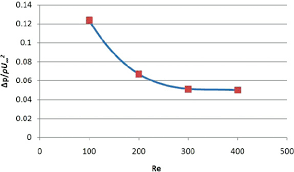The image above represents dimensionless pressure drop.

To compute for the dimensionless pressure drop, five essential parameters are needed and these parameters are Permeability-Thickness Product (kh), Pressure Drop (ΔP), Oil Rate (Qo), Oil Viscosity (μo) and Oil FVF (Bo).

The formula for calculating dimensionless pressure drop:

PD = khΔP / 141.2QoBoμo

Where;

PD = Dimensionless Pressure Drop
kH = Permeability-Thickness Product
ΔP = Pressure Drop
Qo = Oil Rate
μo = Oil Viscosity
Bo = Oil FVF

Let’s solve an example;
Find the dimensionless pressure drop with a permeability-thickness product of 70, a pressure drop of 30, an oil rate of 49, an oil viscosity of 19 and oil FVF of 31.

This implies that;

kh = Permeability-Thickness Product = 20
ΔP = Pressure Drop = 30
Qo = Oil Rate = 49
μo = Oil Viscosity = 19
Bo = Oil FVF = 31

PD = khΔP / 141.2QoBoμo
PD = 20 x 30/141.2 x 49 x 31 x 19
PD = 20 x 30/4075173.19
PD = 600/4075173.19
PD = 0.000147

Therefore, the dimensionless pressure drop is 0.000147 psi.

Calculating the Permeability-Thickness Product when Dimensionless Pressure Drop, Pressure Drop, Oil Rate, Oil Viscosity and Oil FVF.

kh = PD x 141.2QoBoμo / ΔP

Where;

kh = Permeability-Thickness Product
PD = Dimensionless Pressure Drop
ΔP = Pressure Drop
Qo = Oil Rate
μo = Oil Viscosity
Bo = Oil FVF

Let’s solve an example;
Find the permeability-thickness product with a dimensionless pressure drop of 28, a pressure drop of 16, an oil rate of 21, an oil viscosity of 18 and oil FVF of 24 .

This implies that;

PD = Dimensionless Pressure Drop = 28
ΔP = Pressure Drop =16
Qo = Oil Rate = 21
μo = Oil Viscosity = 18
Bo = Oil FVF = 24

kh = PD x 141.2QoBoμo / ΔP
kh = 28 x 141.2 x 21 x 24 x 18 / 16
kh = 35867059.8 / 16
kh = 2241691.2

Therefore, the permeability-thickness product is 2241691.2.

Calculating the Pressure Drop when Dimensionless Pressure Drop, Permeability-Thickness Product, Oil Rate, Oil Viscosity and Oil FVF.

ΔP = PD x 141.2QoBoμo / kh

Where;

ΔP = Pressure Drop
PD = Dimensionless Pressure Drop
kh = Permeability-Thickness Product
Qo = Oil Rate
μo = Oil Viscosity
Bo = Oil FVF

Let’s solve an example;
Find the pressure drop with a dimensionless pressure drop of 38, a permeability-thickness product of 24, an oil rate of 11, an oil viscosity of 8 and oil FVF of 12.

This implies that;

PD = Dimensionless Pressure Drop = 38
kh = Permeability-Thickness Product = 24
Qo = Oil Rate = 11
μo = Oil Viscosity = 8
Bo = Oil FVF = 12

ΔP = PD x 141.2QoBoμo / kh
ΔP = 38 x 141.2 x 11 x 12 x 8 / 24
ΔP = 5666073.6 / 24
ΔP = 236086.4

Therefore, the pressure drop is 236086.4.

Calculating the Oil Rate when Dimensionless Pressure Drop, Permeability-Thickness Product, Pressure Drop, Oil Viscosity and Oil FVF.

Qo = khΔP / PD x 141.2Boμo

Where;

Qo = Oil Rate
PD = Dimensionless Pressure Drop
kh = Permeability-Thickness Product
ΔP = Pressure Drop
μo = Oil Viscosity
Bo = Oil FVF

Let’s solve an example;
Find the oil rate with a dimensionless pressure drop of 60, a permeability-thickness product of 30, a pressure drop of 34, an oil viscosity of 11 and oil FVF of 25.

This implies that;

PD = Dimensionless Pressure Drop = 60
kh = Permeability-Thickness Product = 30
ΔP = Pressure Drop =34
μo = Oil Viscosity = 11
Bo = Oil FVF = 25

Qo = khΔP / PD x 141.2Boμo
Qo = 30 x 34 / 60 x 141.2 x 25 x 11
Qo = 1020 / 2329800
Qo = 4.37e-4

Therefore, the oil rate is 4.37e-4

Calculating the Oil FVF when Dimensionless Pressure Drop, Permeability-Thickness Product, Pressure Drop, Oil Rate and Oil Viscosity.

Bo = khΔP / PD x 141.2Qoμo

Where;

Bo = Oil FVF
PD = Dimensionless Pressure Drop
kh = Permeability-Thickness Product
ΔP = Pressure Drop
Qo = Oil Rate
μo = Oil Viscosity

Let’s solve an example;
Find the oil FVF with a dimensionless pressure drop of 40, a permeability-thickness product of 10, a pressure drop of 7, an oil rate of 3 and oil viscosity of 5.

This implies that;

PD = Dimensionless Pressure Drop = 40
kh = Permeability-Thickness Product = 10
ΔP = Pressure Drop = 7
Qo = Oil Rate = 3
μo = Oil Viscosity = 5

Bo = khΔP / PD x 141.2Qoμo
Bo = 10 x 7 / 40 x 141.2 x 3 x 5
Bo = 70 / 84720
Bo = 8.26e-4

Therefore, the oil FvF is 8.26e-4.

Calculating the Oil Viscosity when Dimensionless Pressure Drop, Permeability-Thickness Product, Pressure Drop, Oil Rate and Oil FVF.

μo = khΔP / PD x 141.2QoBo

Where;

μo = Oil Viscosity
PD = Dimensionless Pressure Drop
kh = Permeability-Thickness Product
ΔP = Pressure Drop
Qo = Oil Rate
Bo = Oil FVF

Let’s solve an example;
Find the oil viscosity with a dimensionless pressure drop of 20, a permeability-thickness product of 9.5, a pressure drop of 4.6, an oil rate of 3.5 and oil FVF of 4.

This implies that;

PD = Dimensionless Pressure Drop = 20
kh = Permeability-Thickness Product = 9.5
ΔP = Pressure Drop = 4.6
Qo = Oil Rate = 3.5
Bo = Oil FVF = 4

μo = khΔP / PD x 141.2QoBo
μo = 9.5 x 4.6 / 20 x 141.2 x 3.5 x 4
μo = 43.7 / 39536
μo = 0.001105

Therefore, the oil viscosity is 0.001105.

Nickzom Calculator – The Calculator Encyclopedia is capable of calculating the dimensionless pressure drop.

To get the answer and workings of the dimensionless pressure drop using the Nickzom Calculator – The Calculator Encyclopedia. First, you need to obtain the app.

You can get this app via any of these means:

To get access to the professional version via web, you need to register and subscribe for NGN 1,500 per annum to have utter access to all functionalities.
You can also try the demo version via https://www.nickzom.org/calculator

Apple (Paid) – https://itunes.apple.com/us/app/nickzom-calculator/id1331162702?mt=8
Once, you have obtained the calculator encyclopedia app, proceed to the Calculator Map, then click on Petroleum under EngineeringNow, Click on Well Testing under Petroleum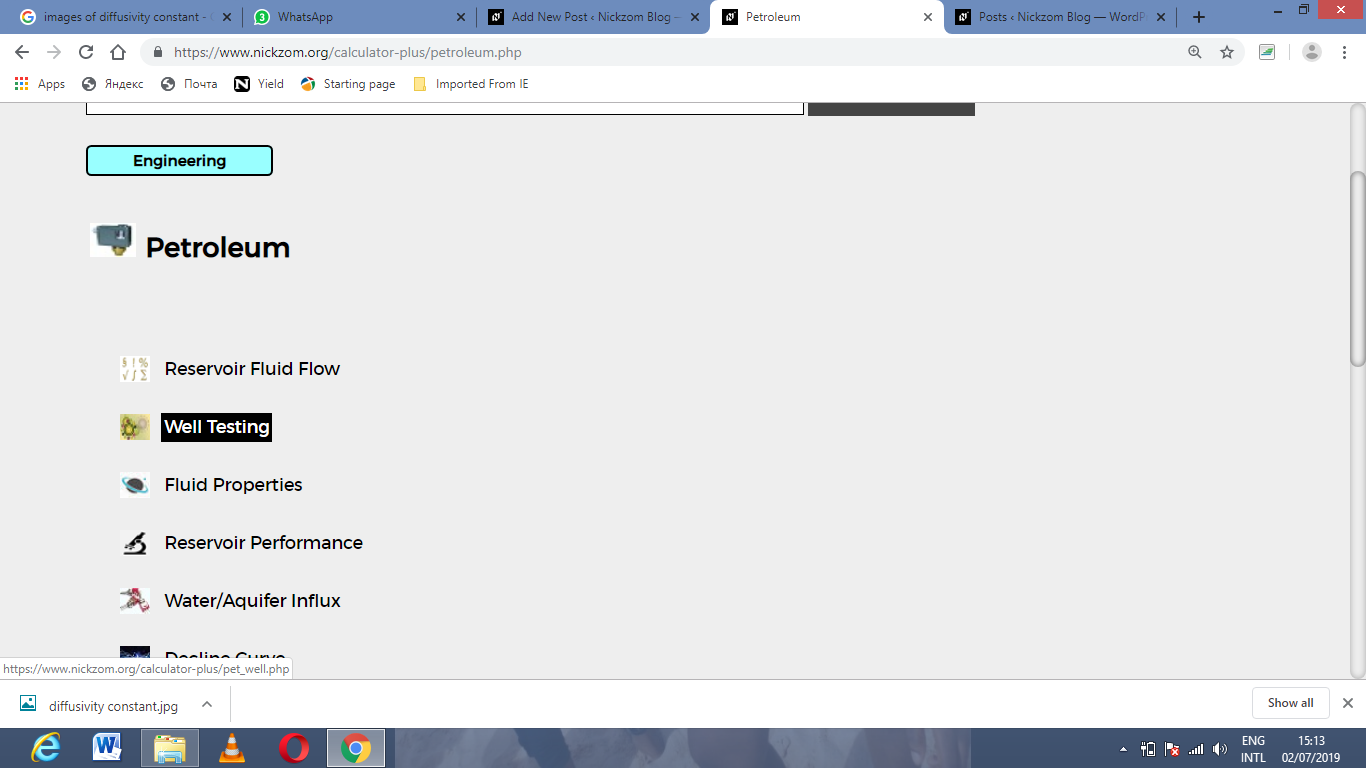Now, Click on Dimensionless Pressure Drop under Well Testing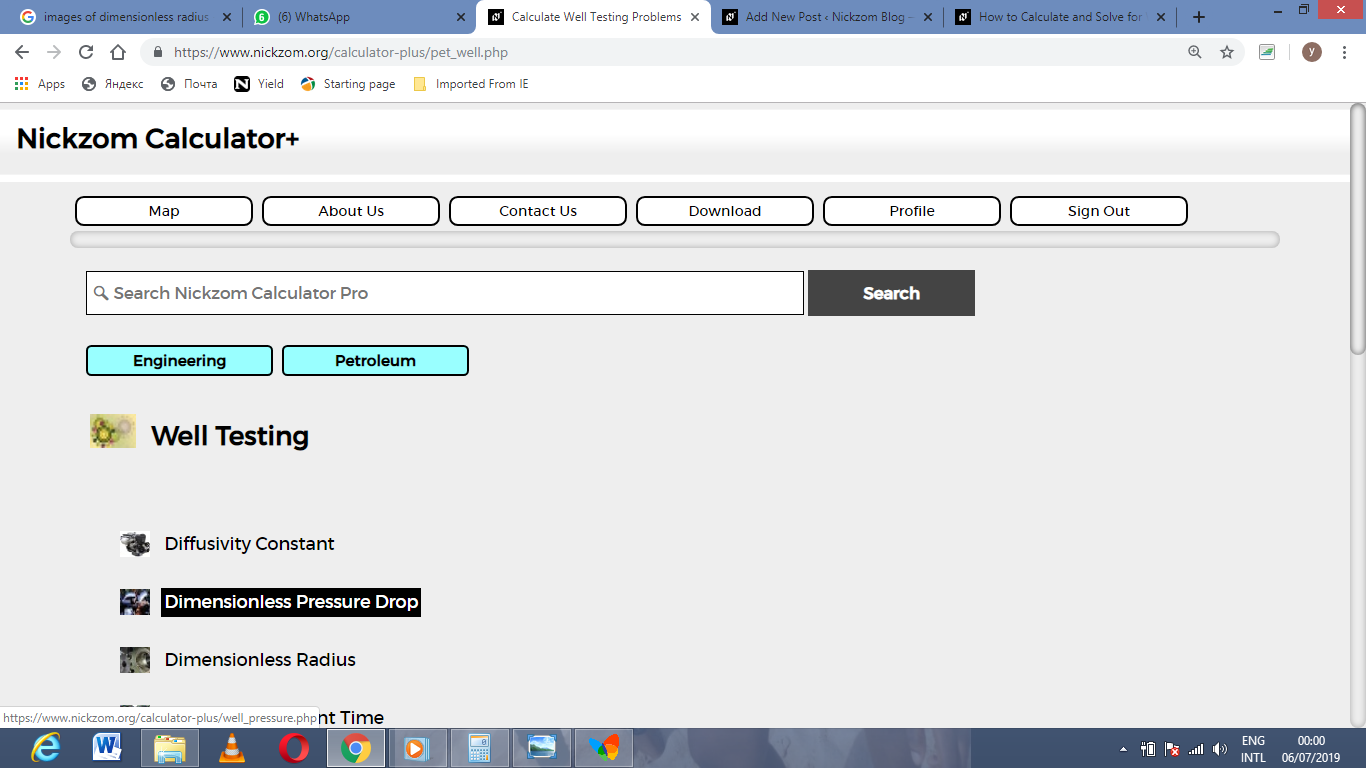The screenshot below displays the page or activity to enter your values, to get the answer for the dimensionless pressure drop according to the respective parameters which are the Permeability-Thickness Product (kh), Pressure Drop (ΔP), Oil Rate (Qo), Oil Viscosity (μo) and Oil FVF (Bo).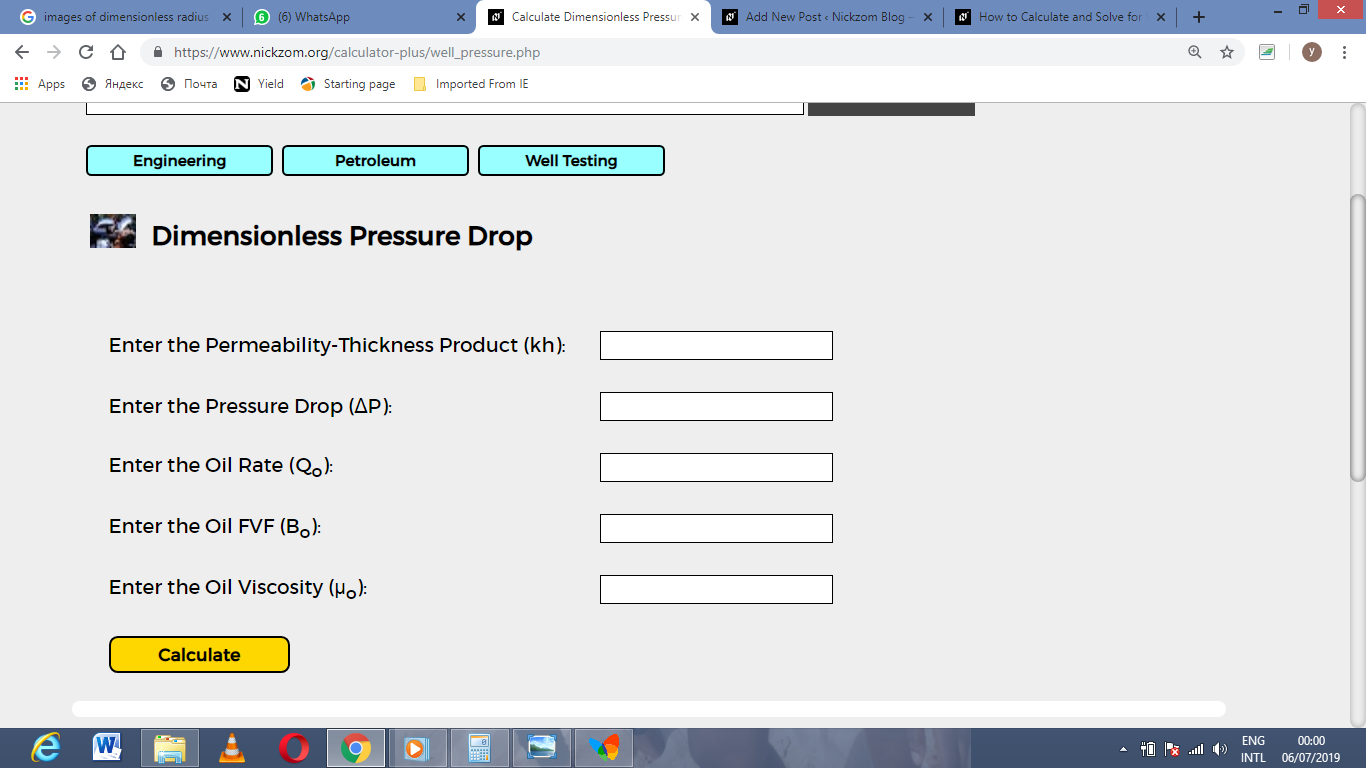Now, enter the values appropriately and accordingly for the parameters as required by the Permeability-Thickness Product (kh) is 20, Pressure Drop (ΔP) is 30, Oil Rate (Qo) is 49, Oil Viscosity (μo) is 19 and Oil FVF (Bo) is 31.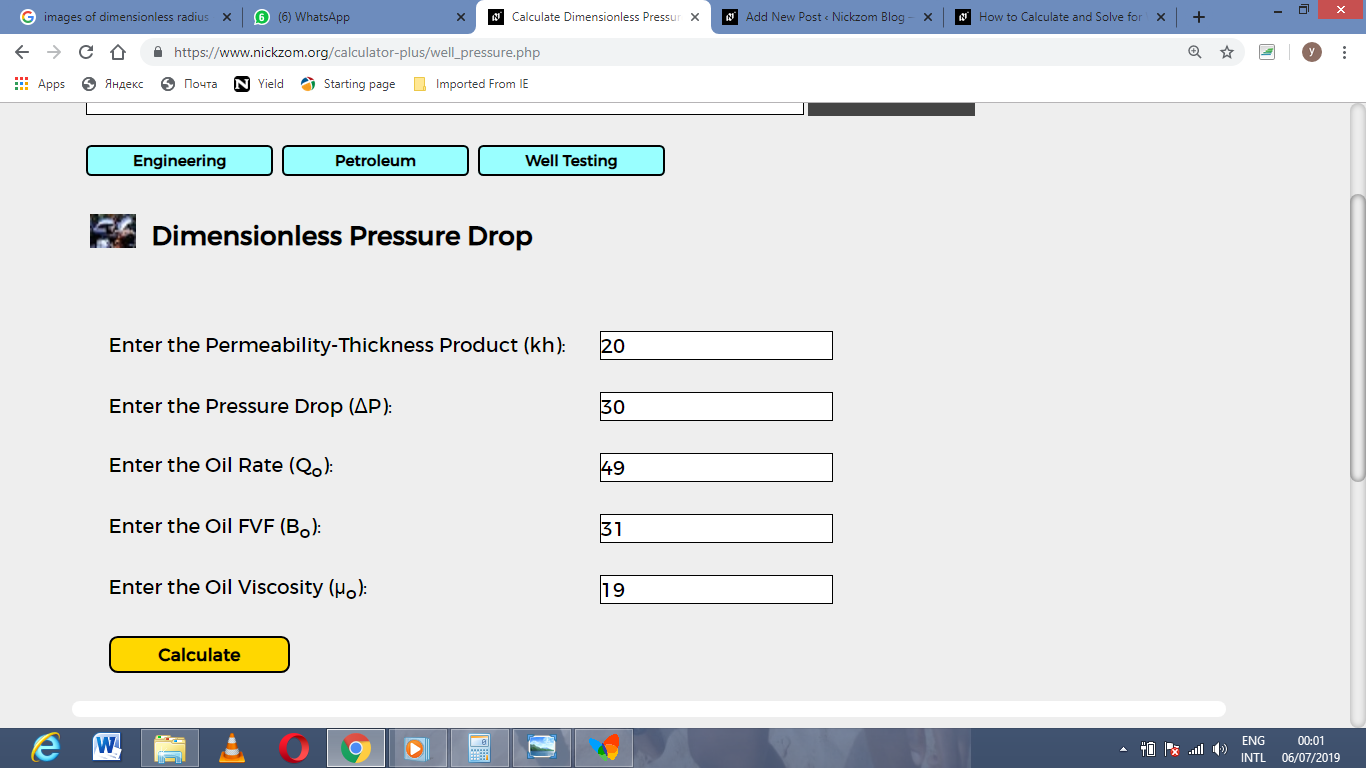Finally, Click on Calculate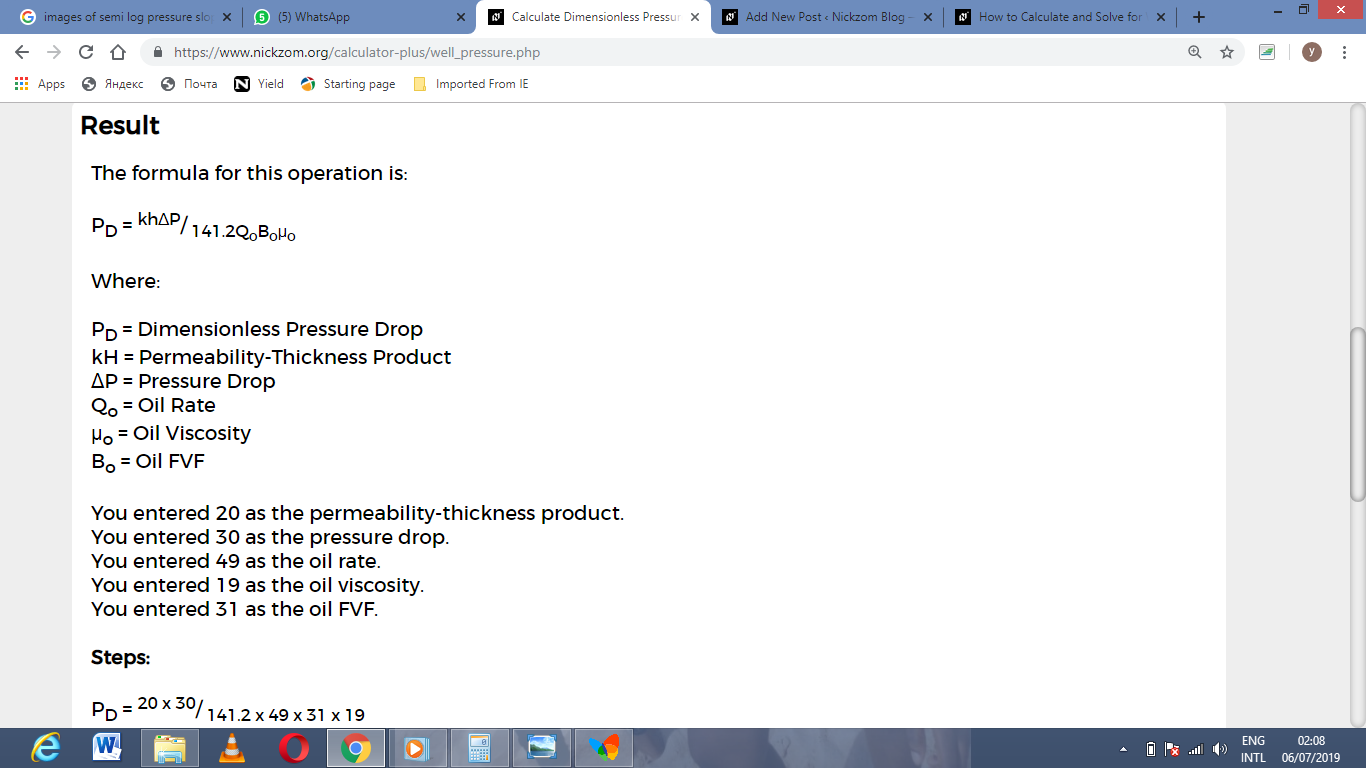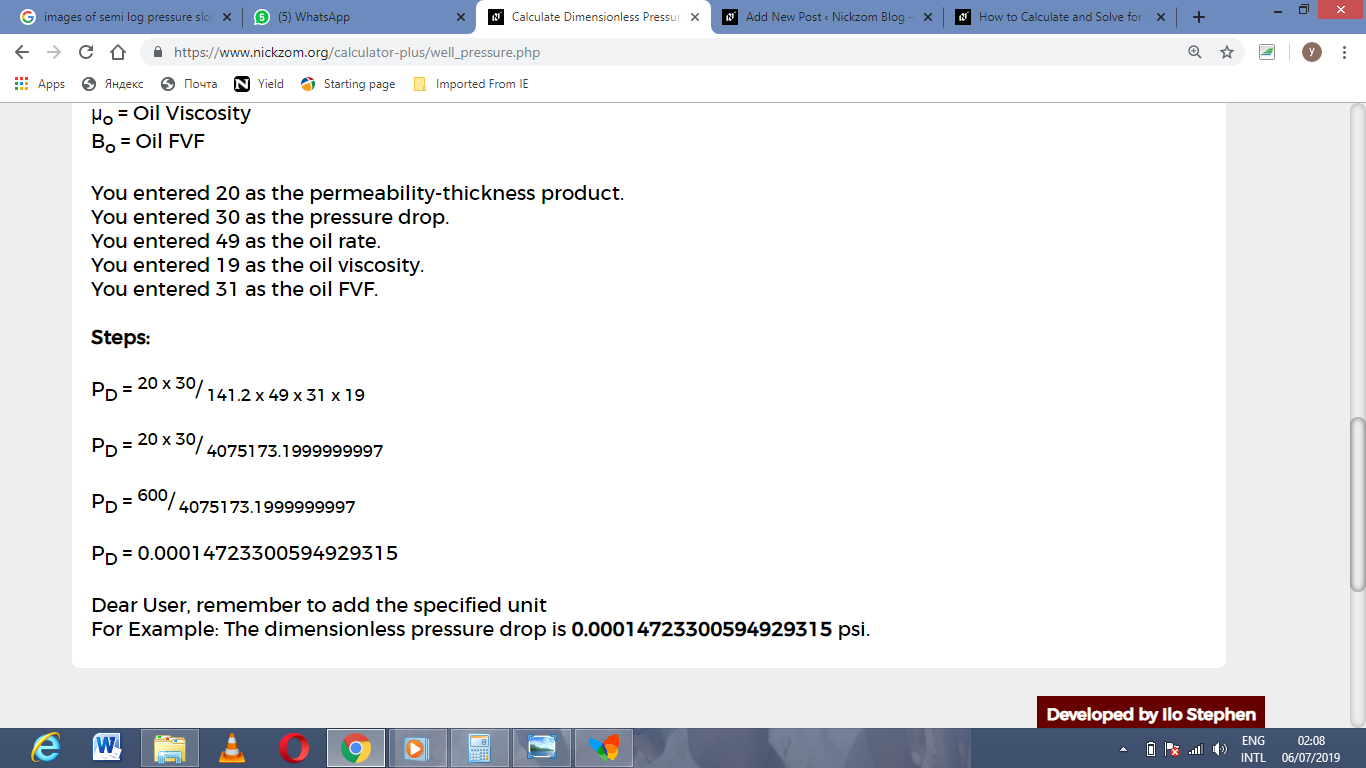As you can see from the screenshot above, Nickzom Calculator– The Calculator Encyclopedia solves for the dimensionless pressure drop and presents the formula, workings and steps too.